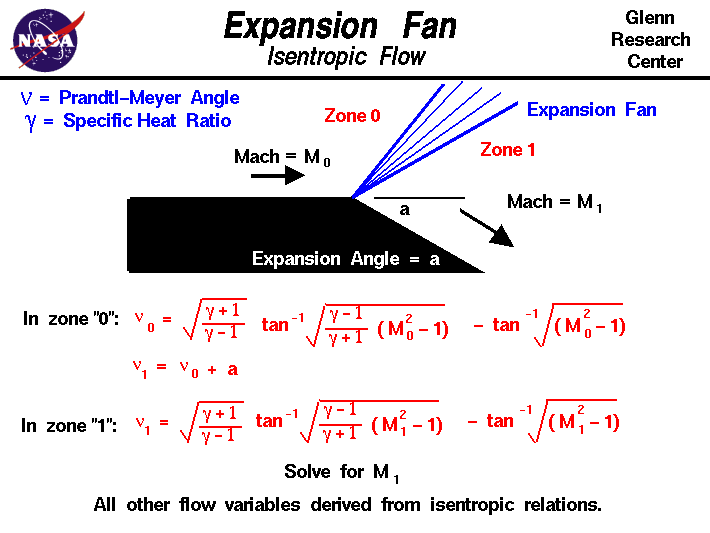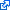+ Text Only Site
+ Non-Flash Version
+ Contact GlennAs an object moves through a gas, the gas molecules are deflected around the object. If the speed of the object is much less than the speed of sound of the gas, the density of the gas remains constant and the flow of gas can be described by conserving momentum and energy. As the speed of the object increases towards the speed of sound, we must consider compressibility effects on the gas. The density of the gas varies locally as the gas is compressed by the object. When an object moves faster than the speed of sound, and there is an abrupt decrease in the flow area, shock waves are generated. If the flow area increases, however, a different flow phenomenon is observed. If the increase is abrupt, we encounter a centered expansion fan. The word "expansion" denotes that the area is increasing. There are some marked differences between shock waves and expansion fans. Across a shock wave, the Mach number decreases, the static pressure increases, and there is a loss of total pressure because the process is irreversible. Through an expansion fan, the Mach number increases, the static pressure decreases and the total pressure remains constant. Expansion fans are isentropic. The calculation of the expansion fan involves the use of the Prandtl-Meyer function. This function is derived from conservation of mass, momentum, and energy for very small (differential) deflections. The Prandtl-Meyer function is denoted by the Greek letter nu on the slide and is a function of the Mach number M and the ratio of specific heats gam of the gas. The physical interpretation of the Prandtl-Meyer function is that it is the angle through which you must expand a sonic (M=1) flow to obtain a given Mach number. To compute an expansion from some other Mach number, we denote the upstream conditions as zone "0" and we calculate the Prandtl-Meyer angle for that Mach number. nu0 = {sqrt[(gam+1)/(gam-1)]} * atan{sqrt[(gam-1)*(M0^2 - 1)/(gam+1)]} - atan{sqrt[M0^2 -1]} where atan is the trigonometric inverse tangent function. It is also written as shown on the slide tan^-1. The meaning of atan can be explained by these two equations: atan(a) = b tan(b) = a We then add the expansion angle a to get the value of the Prandtl-Meyer function in zone "1". nu1 = nu0 + a Then we must iterate (or look up in a table) to obtain the Mach number in zone "1" which gives that value of the Prandtl-Meyer function. nu1 = {sqrt[(gam+1)/(gam-1)]} * atan{sqrt[(gam-1)*(M1^2 - 1)/(gam+1)]} - atan{sqrt[M1^2 -1]} Knowing the Mach number in zone "1" and knowing that the flow is isentropic, we can relate the value of all the flow variables in zone "1" to the variables in zone "0" through the ratio to the total conditions given on the isentropic flow page. The centered expansion shown on this slide is a special case of the distributed expansion. Flow expansions can be generated over a long distance not the sharp edge noted on the slide. Since the flow is isentropic, it is reversible. Under very careful conditions, we can create isentropic compressions which take a high Mach number flow down to low Mach numbers, and increase the static pressure without the loss of total pressure associated with shock waves. Isentropic compression inlets have been designed for high speed flows, but typically remain isentropic only over a narrow operating range. If the free stream Mach number is changed, the isentropic compression waves often coalesce into a shock wave with the accompanying loss in total pressure. Here's a Java program which solves the expansion fan problem. Due to IT security concerns, many users are currently experiencing problems running NASA Glenn educational applets. The applets are slowly being updated, but it is a lengthy process. If you are familiar with Java Runtime Environments (JRE), you may want to try downloading the applet and running it on an Integrated Development Environment (IDE) such as Netbeans or Eclipse. The following are tutorials for running Java applets on either IDE: NetbeansEclipseThis page shows an interactive Java applet for supersonic flow past multiple wedges. The applet shows the shock waves generated by the wedges and the value of the flow variables downstream of the shocks. To study the expansion fan, you can choose from two types of problems: the default problem assumes that you know the angle of expansion and wish to solve for the flow downstream of the expansion; the alternate problem assumes that you have a specified pressure ratio and you wish to determine the angle of the expansion and the other flow variables. Input to the program is made using the sliders, or input boxes at the lower left. To change the value of an input variable, simply move the slider. Or click on the input box, select and replace the old value, and hit Enter to send the new value to the program. Output from the program is displayed in output boxes at the lower right. The flow variables are presented as ratios to the free stream value (0). The graphic at the top shows the expansion surface in red and the initial and terminal waves generated by the surface as lines. The user can move the display by clicking on the graphic, holding down, and dragging the graphic. You can zoom in or out of the graphic by using the slider at the left. If you loose the graphic, click on "Find" to restore the initial display. This simulation was derived from the ShockModeler simulation program. ShockModeler can also be used to study the intersection and reflection of multiple shock waves. If you are an experienced user of this simulator, you can use a sleek version of the program which loads faster on your computer and does not include these instructions. You can also download your own copy of the program to run off-line by clicking on this button: Activities: Guided ToursCompressible Aerodynamics:Shock Waves and Expansions:Multiple Shock Wave Simulator:Navigation ..Beginner's Guide Home Page+ Inspector General Hotline + Equal Employment Opportunity Data Posted Pursuant to the No Fear Act + Budgets, Strategic Plans and Accountability Reports + Freedom of Information Act + The President's Management Agenda + NASA Privacy Statement, Disclaimer, and Accessibility CertificationEditor: Nancy Hall NASA Official: Nancy Hall Last Updated: May 13 2021 + Contact Glenn